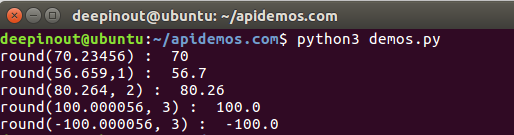# Python round() 函数

## Python round() 函数

Python round() 方法返回浮点数 x 的四舍五入值，准确的说保留值将保留到离上一位更近的一端（四舍六入）。

## Python round() 语法

round( x [, n]  )

## Python round() 参数

• x – 数字表达式。
• n – 表示从小数点位数，其中 x 需要四舍五入，默认值为 0。

## Python round() 示例1

#!/usr/bin/python3

print ("round(70.23456) : ", round(70.23456))
print ("round(56.659,1) : ", round(56.659,1))
print ("round(80.264, 2) : ", round(80.264, 2))
print ("round(100.000056, 3) : ", round(100.000056, 3))
print ("round(-100.000056, 3) : ", round(-100.000056, 3))## Python round() 示例2

>>> round(2.675, 2)
2.67

• 微信订阅
• 回顶